# Many records

In the last lesson, we looked at this DB:

households table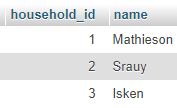doggos table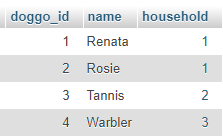There's a one-to-many relationship. A dog can only be in one household, but a household can have many dogs. We represent that by using the primary key of `households` as a foreign key in `doggos`.

We then made a query to find the name of Warbler's household.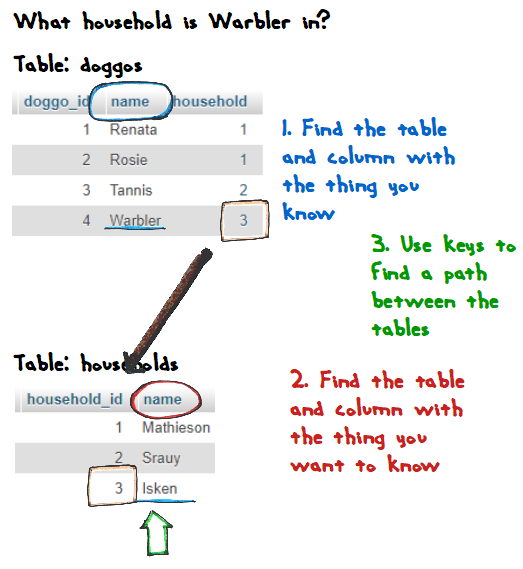The SQL was:

• SELECT households.name
• FROM doggos, households
• WHERE doggos.name = 'Warbler'
•   and doggos.household = households.household_id

This query gave us one result, since Warbler can only be in one household.

### What dogs are in a household?

Let's flip the question around: what dogs are in the Mathieson household? Here's the data again:

households tabledoggos tableYou can see there are two: Renata, and Rosie.

But we don't know how to deal with multiple records coming from the DB.

Time to learn how.

### The SQL

To make the SQL for the query, let's follow the same steps as before.

1. Find the table and column with the thing you know.
2. Find the table and column with the thing you want to know.
3. Use keys to find a path between the tables.

Here's a query template:

• SELECT
• FROM
• WHERE
• ORDER BY

I added an ORDER BY this time, since we'll get multiple records back.

The question is: which dogs are in the Mathieson household?

The first step: Find the table and column with the thing you know.

We know the name of the household. Let's add that to WHERE.

• SELECT
• FROM households
• WHERE households.name = 'Mathieson'
• ORDER BY

Next step: Find the table and column with the thing you want to know.

We want to know the dog's names. That's what we'll sort by, as well.

• SELECT doggos.name
• FROM households, doggos
• WHERE households.name = 'Mathieson'
• ORDER BY doggos.name

OK, now the last step: Use keys to find a path between the tables.

We start in households, and go to doggos. Let's add that link:

• SELECT doggos.name
• FROM households, doggos
• WHERE households.name = 'Mathieson'
•    AND households.household_id = doggos.household
• ORDER BY doggos.name

Here's another way to look at it.

Start in the `households` table, and find the rows with 'Mathiesons' in the `name` field.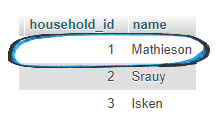Grab the id of that household, and track it to the `household` foreign key in `doggos`.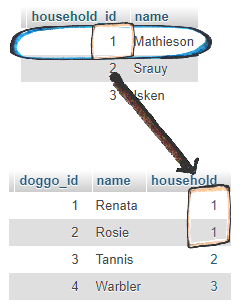Now grab values from the `name` field of those records.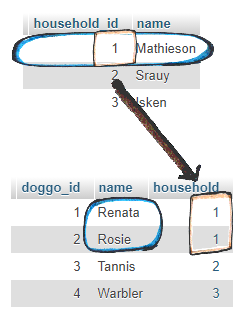### A new HTML tag: ul

We want the output to look like this: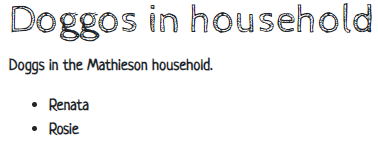A bulleted list of doggos. There's an HTML tag to make lists like that: `ul`, the unordered list tags.

Here's the HTML from the page that made the screenshot:

• <ul>
• <li>Renata</li>
• <li>Rosie</li>
• </ul>

The entire list is between the start and end `ul`s. Each list item is in a pair of `li`s.

### Changing the query PHP

Not much changes in the PHP that runs the query. Here it is. The variable `\$householdName` contains the household the user wants, taken from the end of the URL.

Just a couple of things to note.

1. // Lookup the doggos.
2. if (\$errorMessage == '') {
3.     \$sql = "
4.         SELECT doggos.name as doggo_name
5.         FROM households, doggos
6.         WHERE households.name = :household_name
7.            AND households.household_id = doggos.household
8.         ORDER BY doggos.name;
9.     ";
10.     /** @var PDO \$dbConnection */
11.     \$stmnt = \$dbConnection->prepare(\$sql);
12.     \$result = \$stmnt->execute(['household_name' => \$householdName]);
13.     // Found any records?
14.     if (\$stmnt->rowCount() == 0) {
15.         \$errorMessage = "Sorry, couldn't find doggos in the \$householdName household.";
16.     }
17.     else {
18.         \$rows = \$stmnt->fetchAll();
19.     }
20. }
21. ?><!doctype html>

With this...

• SELECT doggos.name

... would the output field be called `name`, or `doggo.name`? I wasn't sure, so I named it explicitly, with AS. It'll be called `doggo_name`. No guessing.

In the examples were the query would return one row, we used this code:

• \$row = \$stmnt->fetch();

Now we might be many rows back. So we change the code to:

• \$rows = \$stmnt->fetchAll();

`\$rows` instead of `\$row`, and `fetchAll` instead of `fetch`.

Other than that, the query code hasn't changed.

The output code is different, though.

### Output

This is what the query returns for the Mathiesons: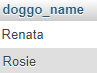We want PHP to turn that into this HTML:

• <ul>
• <li>Renata</li>
• <li>Rosie</li>
• </ul>

Here's some pseudocode:

• Print <ul>
• For each result row:
•   Print <li> doggo_name </li>
• Print </ul>

OK, let's see what this...

• \$rows = \$stmnt->fetchAll();

... actually gives us. I can check with PHPStorm's debugger.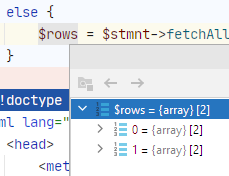It sends back an array.

An array is a list of things, like a column in a worksheet: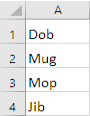The array is in PHP's memory, though, not on a worksheet.

Each array is in a variable. For example, here is an array of goat names: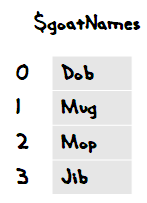​Some goats

`\$goatNames` is the entire array. If we want to get an individual value, we need to use an index. So:

• `\$goatNames` is Dob
• `\$goatNames` is Mug
• `\$goatNames` is Mop
• `\$goatNames` is Jib

In PHP, the indexes can be strings, too. Here's a TMNT array: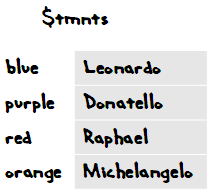​Turtle power!

• `\$tmnts['blue']` is Leonardo
• `\$tmnts['purple']` is Donatello
• `\$tmnts['red']` is Raphael
• `\$tmnts['orange']` is Michelangelo

You can even mix the index types.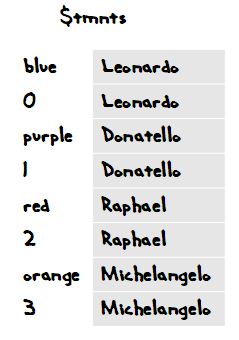​Mixed indexes

This array has eight elements. You can access the elements by mask color, or number.

What this does...

• \$rows = \$stmnt->fetchAll();

... is make `\$rows` an array with two elements, one for each row.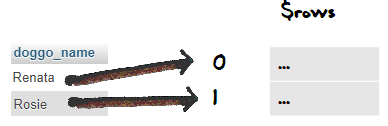Wait, that won't work. What if we'd done a query that sent back more than one field, like:

• SELECT doggos.id, doggos.name...

You'd have to shove id and name into one element. Like trying to put two values into the same Excel cell.

Hmm. You have a point. Here's the goat names from before:​Goat names

We couldn't just shove another value, like goat cuteness rating, into the same cell.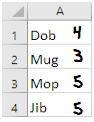​​Goat names and cutenessEthan

In Excel, you'd use another column. So each goat had two a row, with two columns.

Like this. I added column names, too.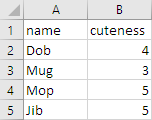​Two columns, with namesYes! Can `\$stmnt->fetchAll()` make that?

Here's that debug shot again:`\$rows` has two elements, one for each row returned by the `SELECT`. Each element is an array of fields.Ray

Whaaaa...

OK, back to the goats for a mo.Let's take Dob's row, just by itself. We could make an array for it, like this: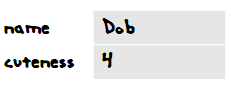Dob's row​

If that array was named, say, `\$x`, `x['name']` would be Dob, and `x['cuteness']` would be 4.

If we made Mug's row into an array: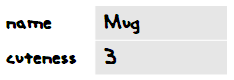Mug's row​

So, we can make an array for each row in...That would give us four arrays, one for each row. Each of those arrays had two elements each.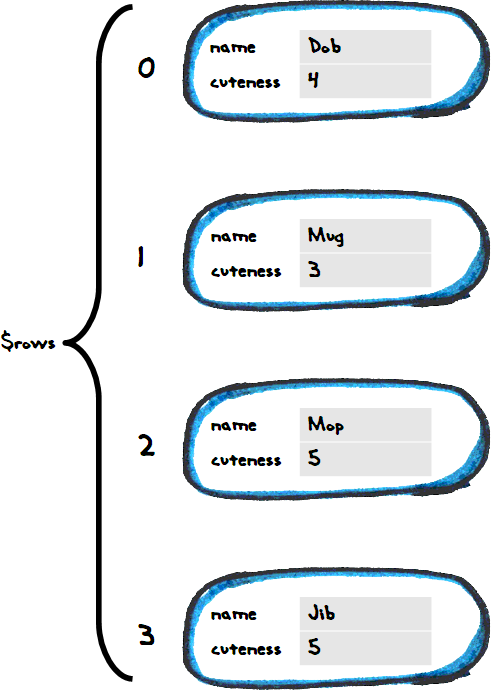​Array of arraysRay

Oh, OK. So, like, `\$rows` is one record, with all of the columns wrapped up inside it.

Right! This is what `fetchAll()` returns, an array of arrays.

You could get at the cuteness of that record like this: `\$rows['cuteness']`.

### Back to the dogs

`\$stmnt->fetchAll()` gave this:`\$rows` is an array of arrays. Open those arrays up, and you see: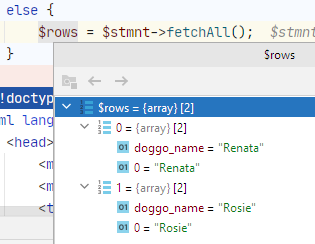PDO's `fetchAll()` gives you two ways to access each field, as you can see, by name, or by number. We'll always use the name, since it makes the code easier to follow.

### Making the list

Here's what we wanted to make:

• <ul>
• <li>Renata</li>
• <li>Rosie</li>
• </ul>

Here's some pseudocode:

• Print <ul>
• For each result row:
•   Print <li> doggo_name </li>
• Print </ul>

Here's the PHP for that:

• print "<ul>\n";
• foreach (\$rows as \$row) {
•     \$doggoName = \$row['doggo_name'];
•     print "<li>\$doggoName</li>\n";
• }
• print "</ul>\n";

This statement...

• \$rows = \$stmnt->fetchAll();

... put an array of records into `\$rows`. We want to go through them one at a time.

This...

• foreach (\$rows as \$row) {
•     Loop body code
• }

... is a loop. `foreach()` goes through the array you give it, once element at a time.

1. The first time through the loop, `\$rows` is put into `\$row`, and the body code is run.
2. The second time through the loop, `\$rows` is put into `\$row`, and the body code is run.
3. The third time through the loop, `\$rows` is put into `\$row`, and the body code is run.
4. ...

Here's the body code:

•     \$doggoName = \$row['doggo_name'];
•     print "<li>\$doggoName</li>\n";

`\$row` is one of the rows. `\$row['doggo_name']` is one field in the row.

### The whole thing

The goal was to let the user ask what doggos were in a household. You can try it, and download the code.

The code:

1. <?php
2. // Connect to the database.
3. require_once 'library/useful-stuff.php';
4. // Show no errors so far.
5. \$errorMessage = '';
6. // Get the household name.
7. \$householdName = getParamFromGet('household_name');
8. \$errorMessage = checkHouseholdName(\$householdName);
9. // Lookup the doggos.
10. if (\$errorMessage == '') {
11.     \$sql = "
12.         SELECT doggos.name as doggo_name
13.         FROM households, doggos
14.         WHERE households.name = :household_name
15.            AND households.household_id = doggos.household
16.         ORDER BY doggos.name;
17.     ";
18.     /** @var PDO \$dbConnection */
19.     \$stmnt = \$dbConnection->prepare(\$sql);
20.     \$isWorked = \$stmnt->execute(['household_name' => \$householdName]);
21.     if (!\$isWorked) {
22.         \$errorMessage = 'Something went wrong with the look up.';
23.     }
24.     if (\$errorMessage == '') {
25.         // Found any records?
26.         if (\$stmnt->rowCount() == 0) {
27.             \$errorMessage = "Sorry, couldn't find doggos in the \$householdName household.";
28.         } else {
29.             \$rows = \$stmnt->fetchAll();
30.         }
31.     }
32. }
33. ?><!doctype html>
34. <html lang="en">
36.         <meta charset="utf-8">
37.         <meta name="viewport" content="width=device-width, initial-scale=1, shrink-to-fit=no">
38.         <title>Doggos in household</title>
40.         <script src="https://code.jquery.com/jquery-3.3.1.min.js"></script>
41.         <script src="https://cdnjs.cloudflare.com/ajax/libs/popper.js/1.14.0/umd/popper.min.js"></script>
42.         <script src="https://stackpath.bootstrapcdn.com/bootstrap/4.1.1/js/bootstrap.min.js"></script>
45.     <body>
46.         <div class="container">
47.             <div class="col">
48.                 <h1>Doggos in household</h1>
49.                 <?php
50.                 if (\$errorMessage != '') {
51.                     print "<p class='alert-danger text-danger m-2 p-2'>\$errorMessage</p>";
52.                 }
53.                 else {
54.                     // There are doggos to show.
55.                     print "<p>Doggos in the \$householdName household.</p>\n";
56.                     print "<ul>\n";
57.                     foreach (\$rows as \$row) {
58.                         \$doggoName = \$row['doggo_name'];
59.                         print "<li>\$doggoName</li>\n";
60.                     }
61.                     print "</ul>\n";
62.                 }
63.                 ?>
64.             </div>
65.         </div>
66.     </body>
67. </html>

Lines 11-32 run a query, and put the results of `fetchAll() into `\$rows@.

Lines 57 to 60 loop over `\$rows`, outputting the `doggo_name` field for each one.

• print "<ul>\n";
• foreach (\$rows as \$row) {
•     \$doggoName = \$row['doggo_name'];
•     print "<li>\$doggoName</li>\n";
• }
• print "</ul>\n";

### Getting real

Our programs are getting closer to those used in real business web apps. The doggo list is a report. Users can ask a question is business terms...

What doggos are in household X?

... and get a result that helps them do their work.

### Exercise

Exercise

Wombat list

Show a list of wombat names. Each one is a link to a page on that wombat's deets. You can try my solution.

Here's the list page: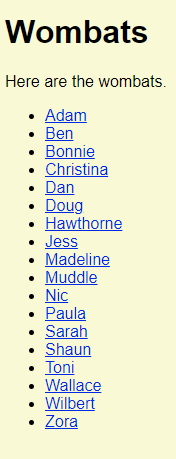The list should be based on data fetched by an SQL query.

Here's a wombat deets page: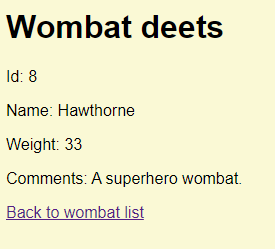Hint: check the HTML produced by the list page. It will show you want you're aiming to produce.

• Put database connection info in a file outside the web root.
• Require that file in a library file.

Here's what my file tree looks like: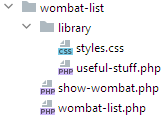Upload the URL of your solution, and a zip of your files. The usual coding standards apply.

### What now?

In the last DB lesson, let's look at many-to-many queries.